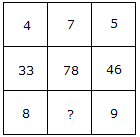# Verbal Reasoning - Character Puzzles - Discussion

### Discussion :: Character Puzzles - Character Puzzles 1 (Q.No.37)

37.

Which one will replace the question mark ?[A]. 12 [B]. 13 [C]. 11 [D]. 10

Explanation:

(4 x 8) + 1 = 33

(5 x 9) + 1 = 46

Similarly, (7 x 11) + 1 = 78.

 Sana said: (May 27, 2012) 4*8=32+1=33 5*9=45+1=46 7*11=77+1=78 Ans:11